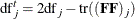# The GAMPL Procedure

### Degrees of Freedom

Let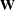be the adjusted weight matrix at convergence, and let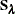be the roughness penalty matrix with selected smoothing parameters. The degrees of freedom matrix is defined as in Wood (2006):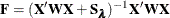Given the adjusted response, the parameter estimate is shown to befor the model without penalization, and the parameter estimate is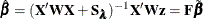with penalization.
is thus the matrix that projects or maps the unpenalized parameter estimates to the penalized ones.

The model degrees of freedom is given as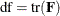And the degrees of freedom for error is given as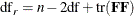For the jth spline term, the degrees of freedom for the component is defined to be the trace of the submatrix ofthat corresponds to parameter estimates
: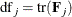The degrees of freedom for the smoothing component test of the jth term is defined similarly as